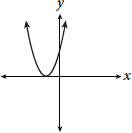# SAT Math Multiple Choice Question 910: Answer and Explanation

### Test Information

Question: 910

10.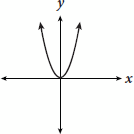If the above graph shows f (x) = 7x2, which of the following is the graph of f (x) = 7x2 + 1?

• A.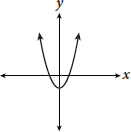• B.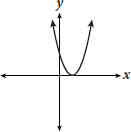• C.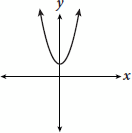• D.